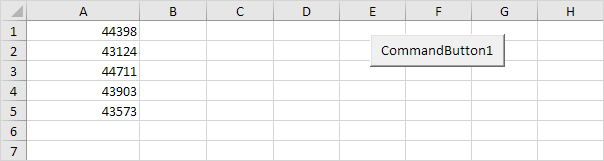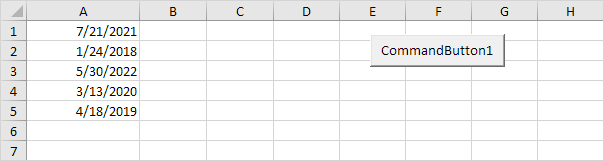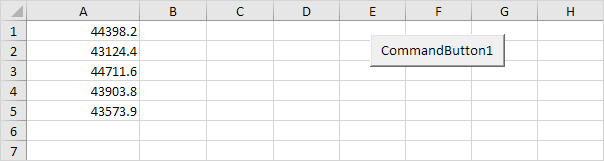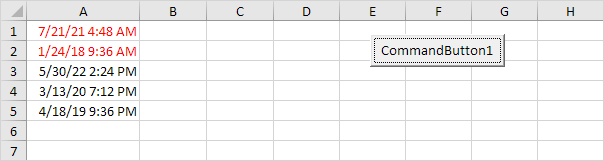# Compare Dates and Times

This example teaches you how to compare dates and times in Excel VBA. Dates and times are stored as numbers in Excel and count the number of days since January 0, 1900. What you see depends on the number format.

1. Enter some numbers in column A.2. These numbers are dates. This is a perfect way to enter some dates without worrying about the Date format. Change the format to Date (Right click on the column A header, Format Cells and choose Date).

Result:Note: Dates are in US Format. Months first, Days Second. This type of format depends on your windows regional settings.

Place a command button on your worksheet and add the following code lines:

3. Declare the variable i of type Integer.

Dim i As Integer

4. Add a For Next loop.

For i = 1 To 5

Next i

5. The Date function returns the current date without the time. Add the following code line to the loop, to highlight all the cells containing the current date (3/13/2020).

If Cells(i, 1).Value = Date Then Cells(i, 1).Font.Color = vbRed

Result:6. Add the following code line to the loop, to highlight all the dates earlier than 04/19/2019.

If Cells(i, 1).Value < DateValue("April 19, 2019") Then Cells(i, 1).Font.Color = vbRed

Result:7. But what about times, we hear you say. They are the decimals. Switch back to General format and change the numbers to decimal numbers.8. Now change the format to 'Date and Time' format.Result:9. If you want to highlight all cells containing the current date, we cannot use the code line at 5 anymore. Why not? Because the numbers in column A are decimal numbers now. Comparing it with Date (a whole number) would not give any match. (It would only give a match with 3/13/2020 at midnight exactly!) The following code line does work:

If Int(Cells(i, 1).Value) = Date Then Cells(i, 1).Font.Color = vbRed

Explanation: we simply use the Int function. The Int function rounds a number down to the nearest integer. This way we can get the dates without the times and compare these dates with Date.

Result:10. Add the following code line to highlight all the cells containing times in the morning.

If (Cells(i, 1).Value - Int(Cells(i, 1).Value)) < 0.5 Then Cells(i, 1).Font.Color = vbRed

Explanation: we only need the decimals so therefore we subtract the integer part. Noon (halfway through the day) is represented as 0.5. Decimals lower than 0.5 are the times in the morning.

Result:Go to Next Chapter: Events